# Basic Arithmetic : Rounding

## Example Questions

### Example Question #1 : Whole Numbers

Round to the nearest hundredth: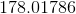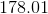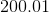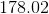Explanation:

The hundredth place is the 2nd number after the decimal. Because the thousandth place is great than 5, we will need to round up to### Example Question #1 : Rounding

Baseballs can only be bought in packages of. If team needs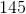balls for the season and always rounds that estimate up, how many packages do they need?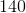Explanation:

1. Round 145 to the nearest 10:

Since there is a 5 in the ones place, 145 rounds up to 150.

2. Divide the rounded number of baseballs by 10 since they come in packages of 10:

150/10=15 packages

### Example Question #1 : Basic Arithmetic

Round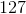to the nearest ten.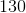Explanation:

To round to the nearest ten, you need to take a look at the ones' place. Since the ones' place is greater than, you will need to round the tens' place up.

So thenis rounded up to.

### Example Question #2 : Rounding

Round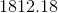to the nearest ones' place.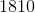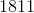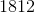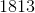Explanation:

To figure out how to round the ones' place, we need to first look at the digit that's to the right of it. If the digit to the right of the ones' place is less than 5, then we leave the ones' place alone.

Since the tenths place is 1, we will leave the ones place alone.

### Example Question #1 : Rounding

Round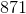to the nearest hundred.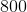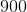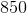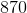Explanation:

To figure out how to round any number, look at the digit to the right of the place you're supposed to be rounding.

Since we want to round the hundreds place, look to the tens place first. If the tens place is greater than 5, then we round up. If the tens place is less than five, then leave the hundreds place alone.

Because the tens place is 7, we will need to round to 900.

### Example Question #1 : Operations With Whole Numbers

What is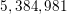rounded to the nearest ten-thousands place?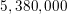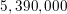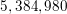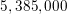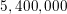Explanation:

An important thing to remember when rounding is knowing which place you're rounding to. In this problem, we our rounding to the ten-thousands place, therefore we need to take a look at two things: 1) which number appears in that place, and 2) what number appears immediately after it:With this number, an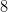appears in the ten-thousands place and a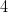appears immediately thereafter. When rounding, we take a look at the number after the place we're rounding to see whether we are rounding up or down. If we have a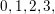orin that place, we will round down, but if we have a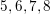or, we will round up. Since in this number we have ain that place, we will round down to. All the places now to the right of thecan be written as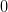s, and our final answer is: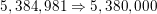### All Basic Arithmetic Resources